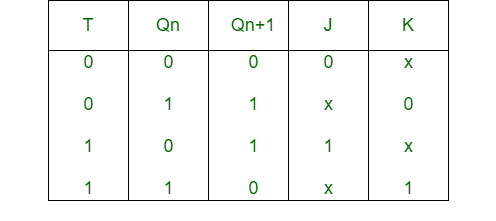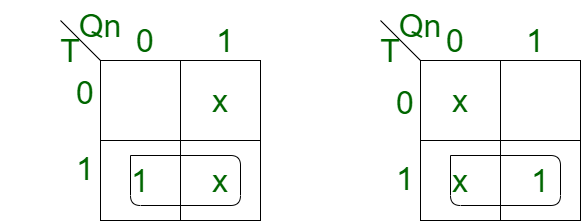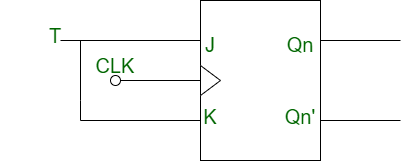# Conversion of J-K Flip-Flop into T Flip-Flop

• Difficulty Level : Medium
• Last Updated : 22 Apr, 2020

Prerequisite – Flip-flop

1. J-K Flip-Flop:
J-K flip-flop is the gated version of Sr flip-flop with an addition of extra input i.e. clock input. It prevents invalid output condition when both the inputs are at the same value.

2. T Flip-Flop:
T flip-flop means Toggle flip-flop. It changes the output on each clock edge and gives an output which is half the frequency of the signal to the input.

Conversion of J-K Flip-Flop into T Flip-Flop:

• Step-1:
Construct the characteristic table of T flip-flop and excitation table of J-K flip-flop.• Step-2:
Using K map, find the boolean expression for J and K in terms of T.```J = T
K = T ```
• Step-3:
Construct the circuit diagram for the conversion of J-K flip-flop into T flip-flop.My Personal Notes arrow_drop_up